Get inspired by the success stories of our students in IIT JAM MS, ISI  MStat, CMI MSc DS.  Learn More

# ISI MStat PSB 2009 Problem 4 | Polarized to NormalThis is a very beautiful sample problem from ISI MStat PSB 2009 Problem 4. It is based on the idea of Polar Transformations, but need a good deal of observation o realize that. Give it a Try it !

## Problem- ISI MStat PSB 2009 Problem 4

Let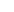and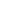be independent and non-negative random variables such that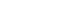and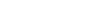. Fix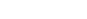. Find the distribution of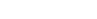.

### Prerequisites

Convolution

Polar Transformation

Normal Distribution

## Solution :

This problem may get nasty, if one try to find the required distribution, by the so-called CDF method. Its better to observe a bit, before moving forward!! Recall how we derive the probability distribution of the sample variance of a sample from a normal population ??

Yes, you are thinking right, we need to use Polar Transformation !!

But, before transforming lets make some modifications, to reduce future complications,

Given,and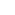is some fixed number in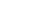, so, let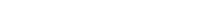.

Hence, we need to find the distribution of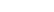. Now, from the given and modified information the joint pdf of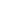and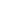are,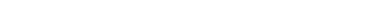Now, let the transformation be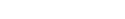,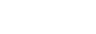, Also, here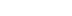Hence,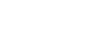Hence, verify the Jacobian of the transformation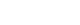.

Hence, the joint pdf of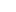andis,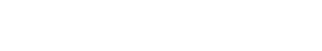,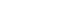.

Yeah, Now it is looking familiar right !!

Since, we need the distribution of, we integrate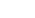w.r.t toover the real line, and we will end up with, the conclusion that,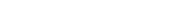. Hence, We are done !!

## Food For Thought

From the above solution, the distribution of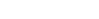is also determinable right !! Can you go further investigating the occurrence pattern of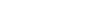??andare the same variables as defined in the question.

Give it a try !!

## Subscribe to Cheenta at Youtube

This is a very beautiful sample problem from ISI MStat PSB 2009 Problem 4. It is based on the idea of Polar Transformations, but need a good deal of observation o realize that. Give it a Try it !

## Problem- ISI MStat PSB 2009 Problem 4

Letandbe independent and non-negative random variables such thatand. Fix. Find the distribution of.

### Prerequisites

Convolution

Polar Transformation

Normal Distribution

## Solution :

This problem may get nasty, if one try to find the required distribution, by the so-called CDF method. Its better to observe a bit, before moving forward!! Recall how we derive the probability distribution of the sample variance of a sample from a normal population ??

Yes, you are thinking right, we need to use Polar Transformation !!

But, before transforming lets make some modifications, to reduce future complications,

Given,andis some fixed number in, so, let.

Hence, we need to find the distribution of. Now, from the given and modified information the joint pdf ofandare,Now, let the transformation be,, Also, hereHence,Hence, verify the Jacobian of the transformation.

Hence, the joint pdf ofandis,,.

Yeah, Now it is looking familiar right !!

Since, we need the distribution of, we integratew.r.t toover the real line, and we will end up with, the conclusion that,. Hence, We are done !!

## Food For Thought

From the above solution, the distribution ofis also determinable right !! Can you go further investigating the occurrence pattern of??andare the same variables as defined in the question.

Give it a try !!

## Subscribe to Cheenta at Youtube

This site uses Akismet to reduce spam. Learn how your comment data is processed.

### Knowledge Partner